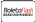Linear Analysis on singular spaces的封面

Linear Analysis on singular spaces

L^2-de Rham-Hodge and L^2-Atiyah-Bott-Lefschetz Theorems on stratified pseudomanifolds有获得代金券的资格
ISBN-13:

978-3-639-85009-3

ISBN-10:
3639850092
EAN:
9783639850093

During the last 30 years stratified pseudomanifolds turned out to be a very reach field of interactions between analysis and topology. The aim of this book is to explore some of these interactions. More precisely the book is divided in two parts. The first half is dedicated to the relationships between the L^2 de Rham cohomology, Hodge cohomology (both associated to a suitable incomplete riemannian metric) and the intersection cohomology of a stratified pseudomanifold. In particular the question of the Poincaré duality for the L^2 cohomology associated to an incomplete metric is discussed. The second half concerns the generalization of the well known theorem of Atiyah-Bott for elliptic complexes over a closed manifolds to the case of elliptic complexes of cone differential operators over a manifold with conical singularities.

https://www.edizioni-ai.com/

Francesco Bei

108

2013-11-06

206.61 R\$

Stratified pseudomanifolds, L2 Cohomology, Hodge cohomology, Intersection cohomology, fixed point formulas, elliptic complexes链接